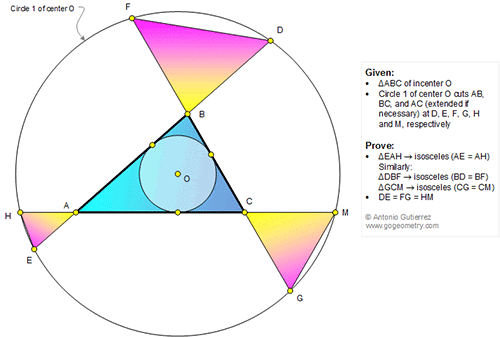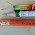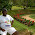## Wednesday, August 9, 2017

### Geometry Problem 1340: Triangle, Incenter, Concentric Circles, Isosceles Triangles, Congruence

Geometry Problem. Post your solution in the comment box below.
Level: Mathematics Education, High School, Honors Geometry, College.

Details: Click on the figure below.1.Let the Radius of Circle 1 be R and the incircle of Trianlge ABC be r
drop _|_lars from O to AB, AC and BC. Denote the points as X, Y & Z
We have OX=OY=OZ=r
Join O to D and consider the right trianlge OXD.
We have XD^2+OX^2 = R^2
=> XD^2 = R^2-r^2 --------------(1)
Similary join OM and consider the right triangle OYM
we have YM^2=R^2-r^2--------------(2)
From (1) and (2) we can say DX = MY and also we have AX = AY (Tangents to the incircle)
=> DA = MA ----------(3)
As HA.AM = EA.AD
=> HA=EA (HAE is isosceles)
Also We can say the chords AM,ED & GF are equal in length

1.I will include your conclusion DE = FG = HM. Thanks.

2.If tr AEH is isosceles then tr ADM is also isosceles since HEDM forms a cyclic quad. This is what we'll show.

Let the tangent point on AB be X and the tangent on AC be Y.
1. tr OXD is congruent to tr OYM since OM = OD (larg radius) and OX=OY (small radius) and they are right triangles. So XD = YM
2. Similarly tr AXO is congruent to AYO by 2 sides (1 common and the radius) and being a right tr. so AX = AY.
3. Add those together AD = AM so tr. ADM is isosceles.
4. As noted from the cyclic quad tr AEH must be also.

This can be repeated for the other 2 sides.

3.Problem 1340
Suppose that a recorded circle tangents to P, Q, R on the sides AC, CB, BA respectively.
Then ER=RD=HP=PM=GQ=QF(OP=OR=OQ). But AR=AP so AH=AE.
Similar BD=BF and CM=CG.
APOSTOLIS MANOLOUDIS 4 HIGH SCHOOL OF KORYDALLOS PIRAEUS GREECE

4.In Circle 1, ED, HM and FG are equidistant from the Centre O and are hence equal.

If the tangent point on ED is X and on HM is Y then EX = HY and because AX = AY, AH = AE

Similarly BF = BD and CM = CG

Sumith Peiris
Moratuwa
Sri Lanka